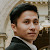Dual Decomposition with Accelerated First-Order Scheme for Discrete Markov Random Fields Optimization

1 post in this topic

This is a little part of the work I did during my Master internship at the Center for Visual Computing, CentraleSupélec, under the supervision of Prof. Nikos Paragios. The work has not been completed since I switched to a more important project, but I think will come back to this topic someday in the near future.

I am sharing it here because I think it may interest some people.

Comments and questions are welcome!

SmoothingDualDecomposition.pdf

2 people like this

Create an account or sign in to comment

You need to be a member in order to leave a comment

Create an account

Sign up for a new account in our community. It's easy!

Register a new account

• Related Content

• By Khue
In this topic I would like to discuss everything about Linear Assignment Problems (LAPs). Another important class of assignment problems, that is Quadratic Assignment Problems, will be discussed later in another topic.
Let's begin with the classic LAP, defined as follows (Wikipedia):
This problem is also called Linear Sum Assignment Problem (LSAP), since the objective function is a sum, to be differentiated with other types of assignment problems such as the Linear Bottleneck Assignment Problem (LBAP). These problems will also be discussed later in this topic. When we say LAP without specification, we refer to the LSAP.
The formal mathematical definition of the LAP is the following.
Problem. (LAP) Given a matrix $C = (c_{ij})_{1\le i,j \le n} \in \mathbb{R}^{n\times n}$, called cost matrix, where $n$ is a given positive integer.
\begin{align} \mbox{minimize}\quad & \sum_{i=1}^n \sum_{j=1}^n c_{ij}x_{ij} \\ \mbox{subject to}\quad & \sum_{i=1}^n x_{ij} = 1 \quad \forall j, \\ & \sum_{j=1}^n x_{ij} = 1 \quad \forall i,\\ & x_{ij} \in \set{0,1} \quad\forall i,j. \end{align}
It is straightforward to see that this problem is equivalent to finding a perfect matching of a bipartite graph, thus it is also known as the bipartite minimum cost perfect matching problem.
It is well-known that the LP relaxation of the above integer program (i.e. the integral constraints $x_{ij} \in \set{0,1}$ are relaxed to $x_{ij} \ge 0$) has optimal solutions at extreme points, thus the LP relaxed problem and the original integer program are equivalent.
• By Khue
In this topic we will discuss Nikos Komodakis's work on the approximate primal-dual method for solving MRF optimization. I will describe in this topic 4 algorithms: PD1, PD2, PD3 and FastPD.
The MRF optimization (or MAP Inference) problem:
\begin{equation}
\begin{aligned}
&\text{minimize}  \quad    && \sum_{p\in V}\sum_{l\in L} \theta_p(l)x_p(l) + \sum_{pq\in E}\sum_{(l,l')\in L^2} \theta_{pq}(l,l')x_p(l)x_q(l')\\
&\text{subject to} \quad    && \sum_{l\in L} x_p(l) = 1 \quad \forall p\in V,\\
& &&\sum_{l'\in L} x_{pq}(l,l') = x_p(l) \quad\forall pq\in E, l\in L, \\
& &&\sum_{l\in L} x_{pq}(l,l') = x_q(l') \quad\forall pq\in E, l'\in L, \\
& &&x_p(l)\in\set{0,1}, x_{pq}(l,l')\in\set{0,1}\quad\forall pq\in E, l\in L, l'\in L.
\end{aligned}
\end{equation}
Replacing the integral constraints in the above problem by $x_p(l)\ge 0, x_{pq}(l,l')\ge 0$ we get its the LP-relaxation. The dual of this LP-relaxation is given by:
\begin{align}
\mbox{maximize}\quad & \sum_{p\in V}\min_{a\in L}h_p(a) \label{eq:dual-objective}\\
\mbox{subject to}\quad &y_{pq}(a) + y_{qp}(b) \le \theta_{pq}(a,b) \quad \forall pq\in E, a\in L,b\in L, \label{eq:dual-feasibility}\end{align}
where the function $h_p(\cdot)$ is defined by $$h_p(a) = \theta_p(a) + \sum_{q:pq\in E} y_{pq}(a).$$
Denote by $x_p$ the chosen label for the node $p$.
...

PD1 Algorithm
This algorithm, at termination, gives a primal-dual pair $(\mathbf{x},\mathbf{y})$ satisfying ($\mathbf{x}$ is always chosen as a labeling, thus primal feasibility always holds and hereafter we will not state it explicitly) :
Relaxed dual feasibility:
\begin{equation}y_{pq}(a) \le \frac{1}{2}\theta_{pq}^{\mathrm{min}}\quad\forall pq\in E,a\in L. \label{eq:relaxed-dual-feasibility} \end{equation}
Clearly, this implies dual feasibility, i.e. \eqref{eq:dual-feasibility}. Relaxed complementary slackness conditions: \begin{align} h_p(x_p) &= \min_ah_p(a) \quad \forall p\in V \label{eq:primal-csc-p} \\ y_{pq}(x_p) + y_{qp}(x_q) &\ge \frac{1}{f_\mathrm{app}} \theta_{pq}(x_p,x_q) \quad \forall pq\in E. \label{eq:relaxed-primal-csc-pq} \end{align} During its iterations, the algorithm ensures all the above conditions, except \eqref{eq:primal-csc-p}, which will be satisfied at the end.
Dual variables update
Given $(\mathbf{x},\mathbf{y})$ satisfying \eqref{eq:relaxed-dual-feasibility} and \eqref{eq:relaxed-primal-csc-pq}. If $(\mathbf{x},\mathbf{y})$ also satisfy \eqref{eq:primal-csc-p} then we obtain an approximate solution. Otherwise, we will be able to increase the dual objective \eqref{eq:dual-objective} as follows.
Since \eqref{eq:primal-csc-p} does not hold for all $p$, there exists a non-empty set $V_0 \subseteq V$ of nodes $p$ where $h_p(x_p) > \min_ah_p(a)$.